# Points Lines and Planes Unit 1 Lesson 2

• Slides: 46Points Lines and Planes Unit 1 Lesson 2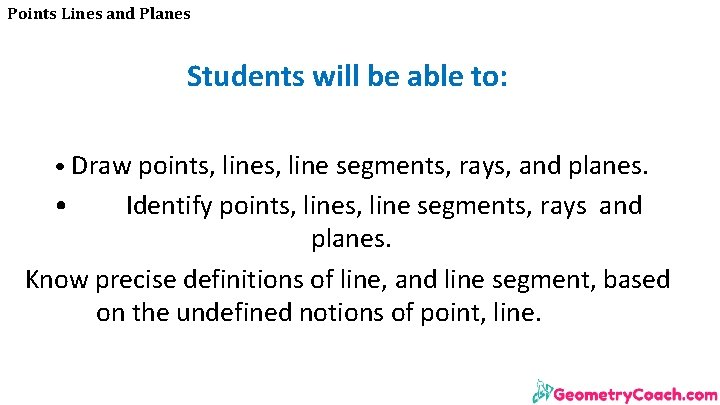Points Lines and Planes Students will be able to: • Draw points, line segments, rays, and planes. • Identify points, line segments, rays and planes. Know precise definitions of line, and line segment, based on the undefined notions of point, line.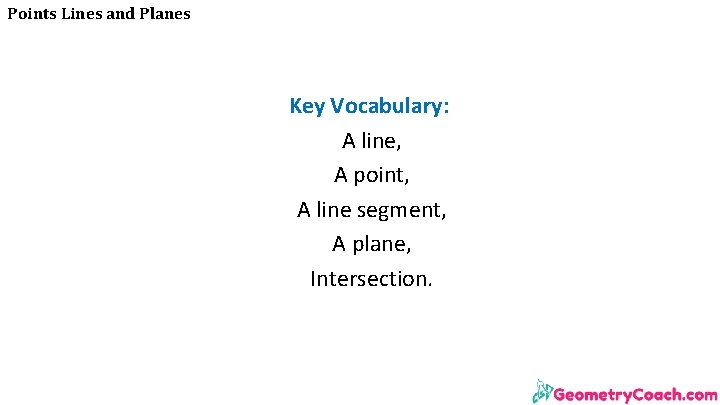Points Lines and Planes Key Vocabulary: A line, A point, A line segment, A plane, Intersection.Points Lines and Planes • In geometry, some words, such as point, line, and plane, are undefined terms. Although these words are not formally defined, it is important to have general agreement about what each word means. • A point has no dimension. • It is usually represented by a small dot and named by a capital letter.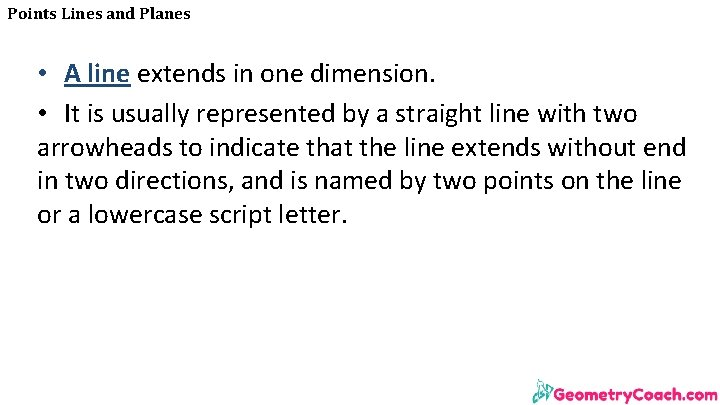Points Lines and Planes • A line extends in one dimension. • It is usually represented by a straight line with two arrowheads to indicate that the line extends without end in two directions, and is named by two points on the line or a lowercase script letter.Points Lines and Planes • A plane extends in two dimensions. • It is usually represented by a shape that looks like a tabletop or wall. • You must imagine that the plane extends without end, even though the drawing of a plane appears to have edges, and is named by a capital script letter or 3 noncollinear points.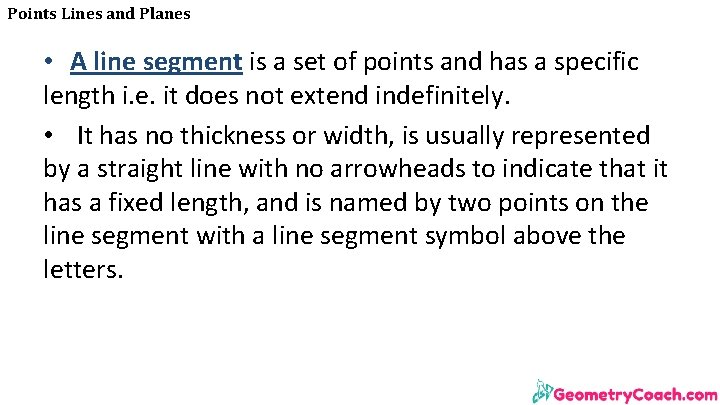Points Lines and Planes • A line segment is a set of points and has a specific length i. e. it does not extend indefinitely. • It has no thickness or width, is usually represented by a straight line with no arrowheads to indicate that it has a fixed length, and is named by two points on the line segment with a line segment symbol above the letters.Points Lines and Planes • A ray is a set of points and extends in one dimension in one direction (not in two directions). It has no thickness or width, is usually represented by a straight line with one arrowhead to indicate that it extends without end in the direction of the arrowhead, and is named by two points on the ray with a ray symbol above the letters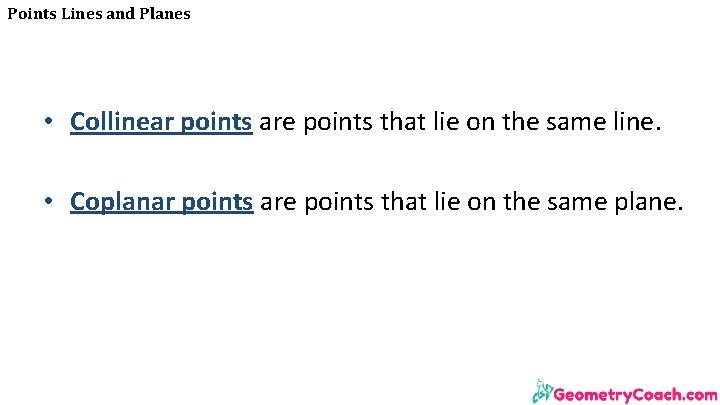Points Lines and Planes • Collinear points are points that lie on the same line. • Coplanar points are points that lie on the same plane.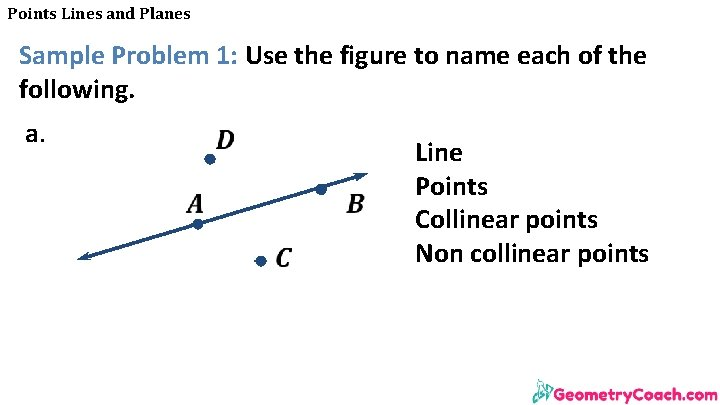Points Lines and Planes Sample Problem 1: Use the figure to name each of the following. a. Line Points Collinear points Non collinear pointsPoints Lines and Planes Sample Problem 1: Use the figure to name each of the following. a.Points Lines and Planes Sample Problem 1: Use the figure to name each of the following. b. Line segment PointsPoints Lines and Planes Sample Problem 1: Use the figure to name each of the following. b.Points Lines and Planes Sample Problem 1: Use the figure to name each of the following. c. Plane Ray Points Coplanar points Non coplanar pointsPoints Lines and Planes Sample Problem 1: Use the figure to name each of the following. c.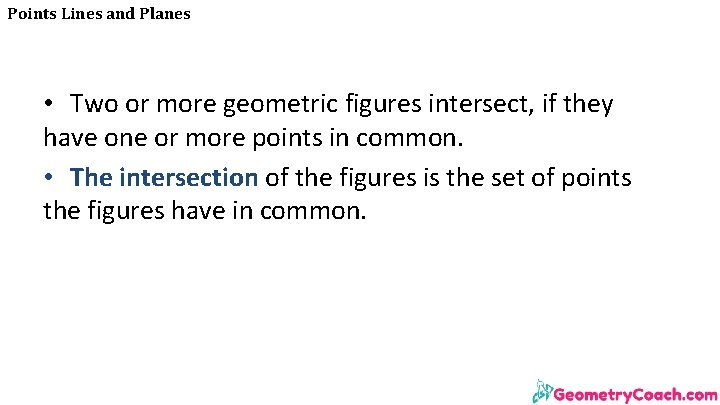Points Lines and Planes • Two or more geometric figures intersect, if they have one or more points in common. • The intersection of the figures is the set of points the figures have in common.Points Lines and Planes • Postulate 1 -1 Through any two points there is exactly one line. • Postulate 1 -2 If two distinct lines intersect, then they intersect in exactly one point. • Postulate 1 -3 If two distinct planes intersect, then they intersect in exactly one line. • Postulate 1 -4 Through any three non collinear points there is exactly one plane. •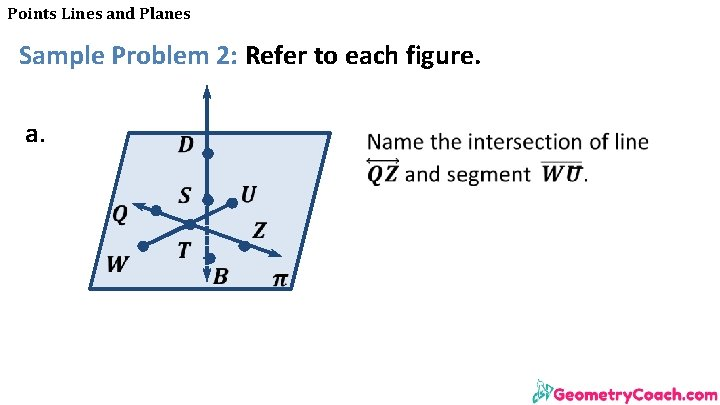Points Lines and Planes Sample Problem 2: Refer to each figure. a.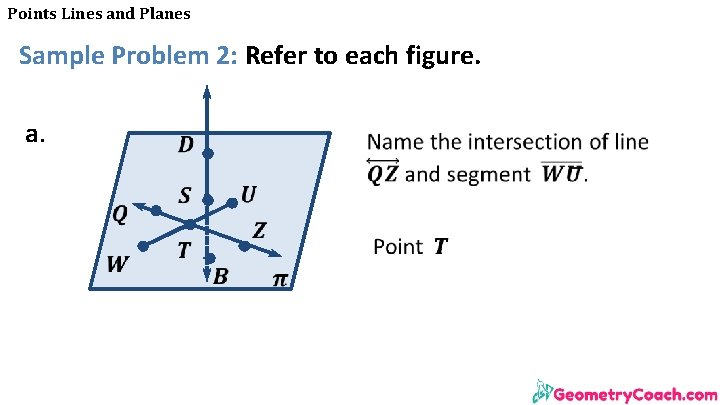Points Lines and Planes Sample Problem 2: Refer to each figure. a.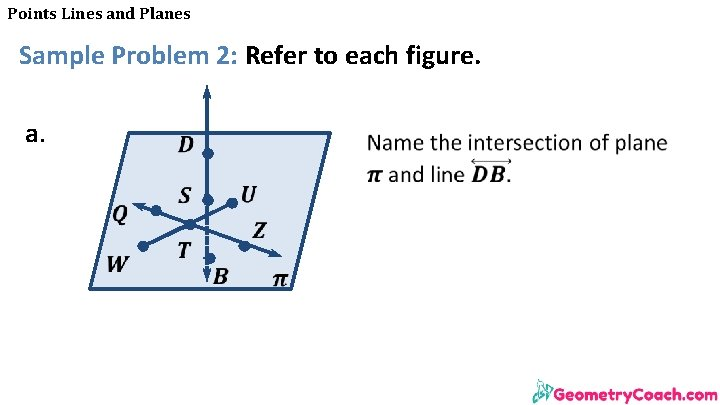Points Lines and Planes Sample Problem 2: Refer to each figure. a.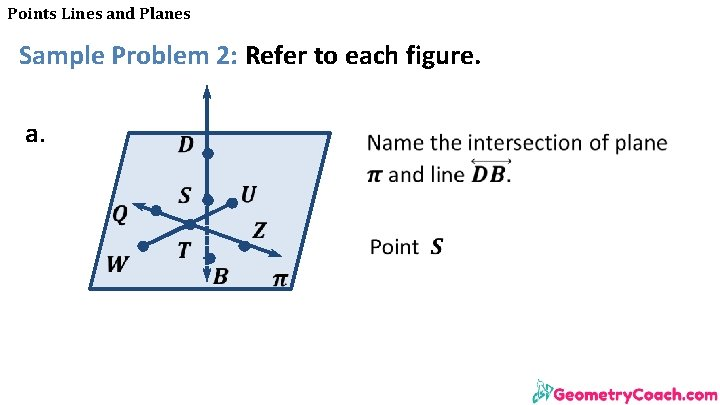Points Lines and Planes Sample Problem 2: Refer to each figure. a.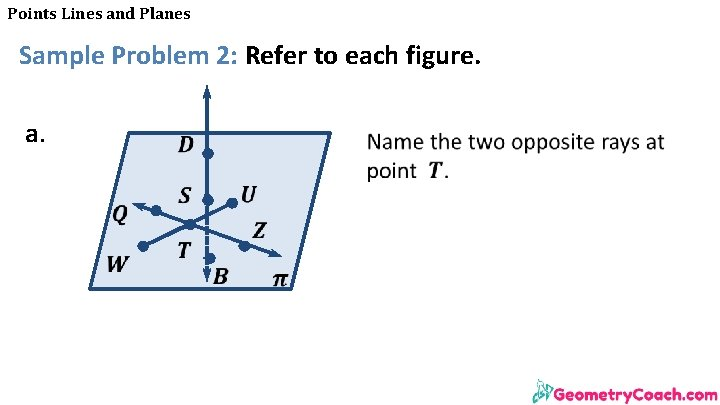Points Lines and Planes Sample Problem 2: Refer to each figure. a.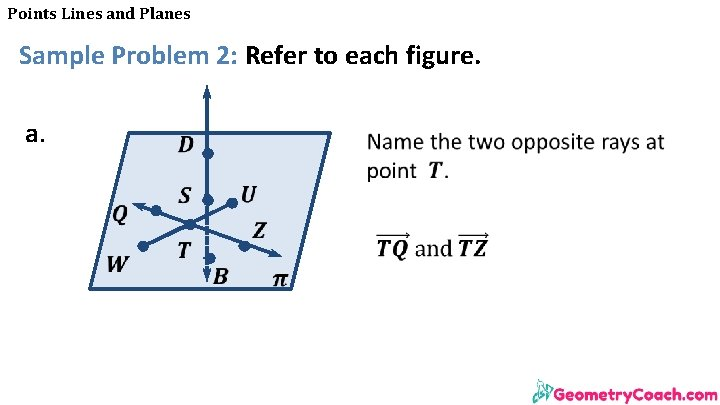Points Lines and Planes Sample Problem 2: Refer to each figure. a.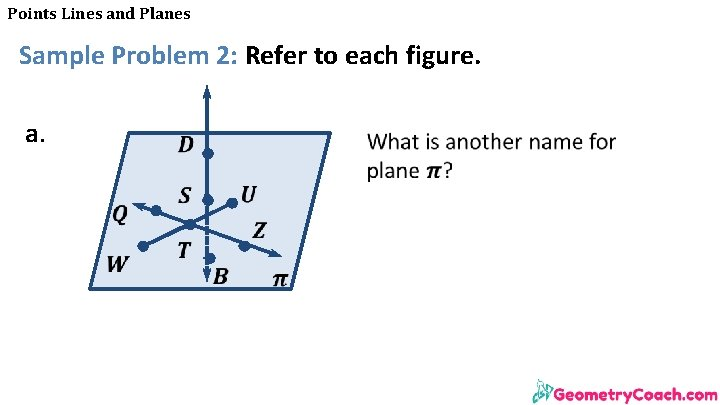Points Lines and Planes Sample Problem 2: Refer to each figure. a.Points Lines and Planes Sample Problem 2: Refer to each figure. a.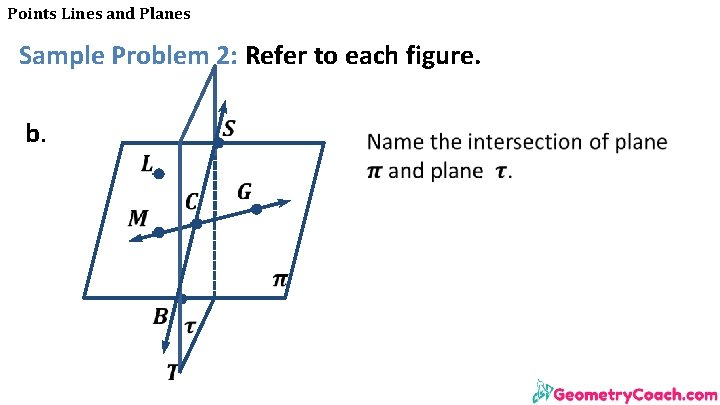Points Lines and Planes Sample Problem 2: Refer to each figure. b.Points Lines and Planes Sample Problem 2: Refer to each figure. b.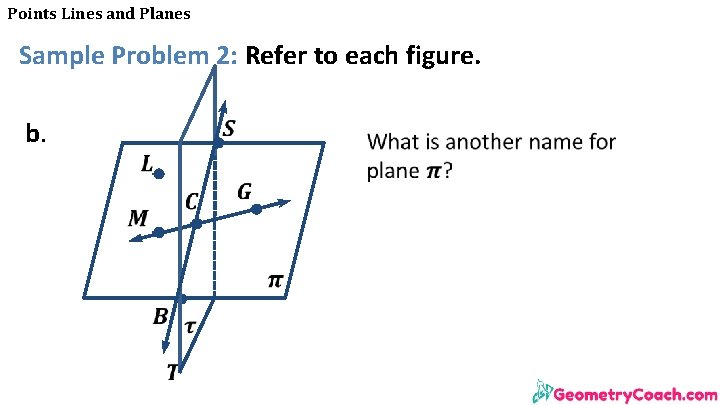Points Lines and Planes Sample Problem 2: Refer to each figure. b.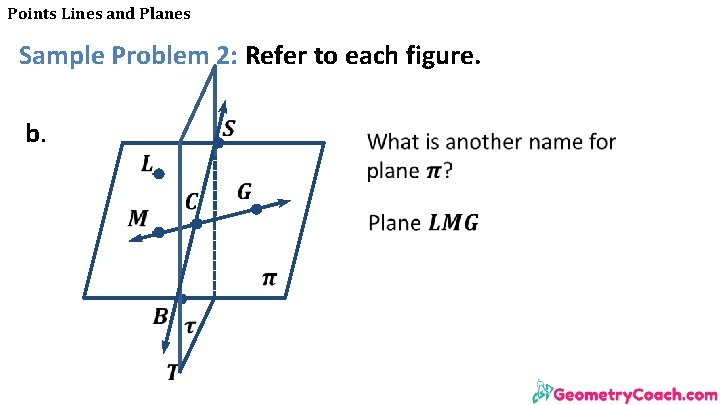Points Lines and Planes Sample Problem 2: Refer to each figure. b.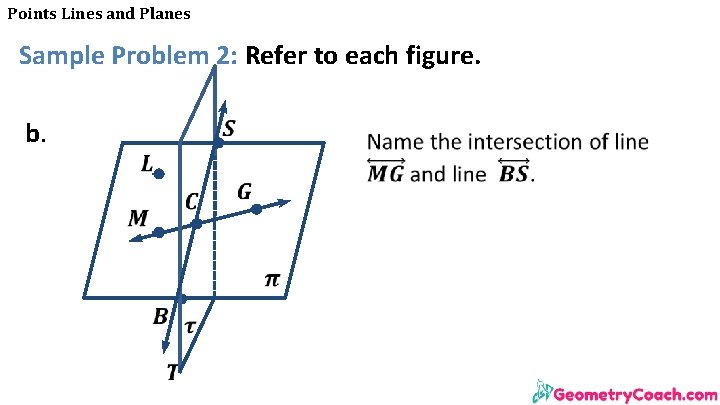Points Lines and Planes Sample Problem 2: Refer to each figure. b.Points Lines and Planes Sample Problem 2: Refer to each figure. b.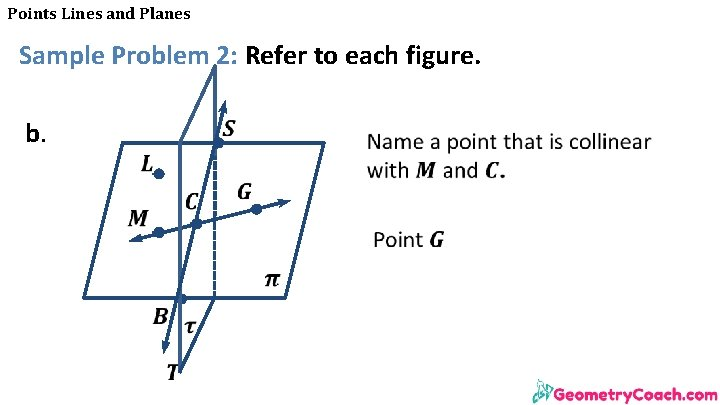Points Lines and Planes Sample Problem 2: Refer to each figure. b.Points Lines and Planes Sample Problem 2: Refer to each figure. c.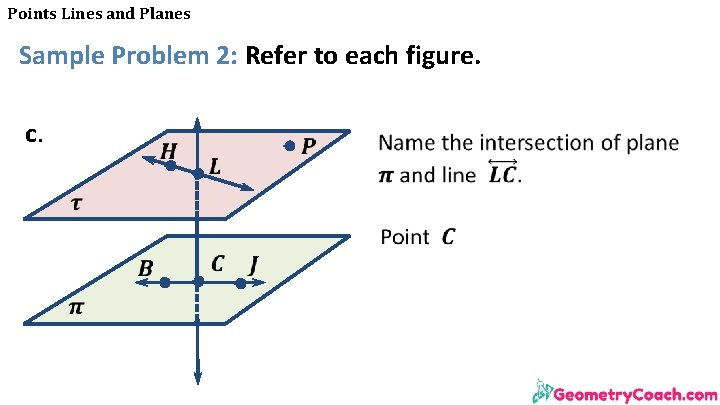Points Lines and Planes Sample Problem 2: Refer to each figure. c.Points Lines and Planes Sample Problem 2: Refer to each figure. c.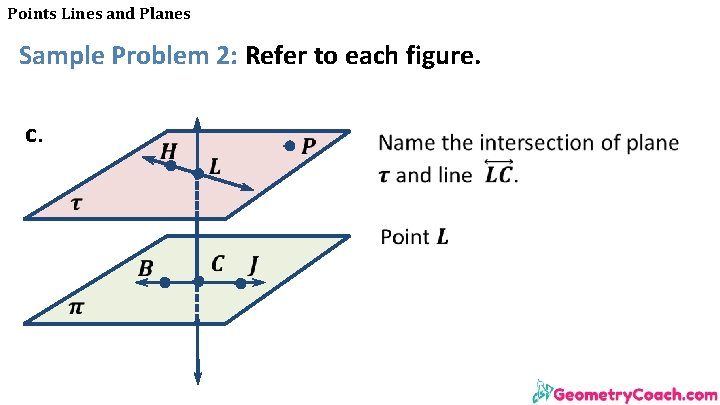Points Lines and Planes Sample Problem 2: Refer to each figure. c.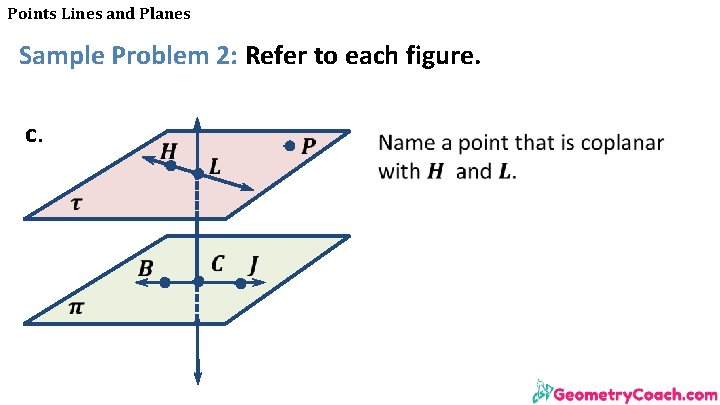Points Lines and Planes Sample Problem 2: Refer to each figure. c.Points Lines and Planes Sample Problem 2: Refer to each figure. c.Points Lines and Planes Sample Problem 2: Refer to each figure. c.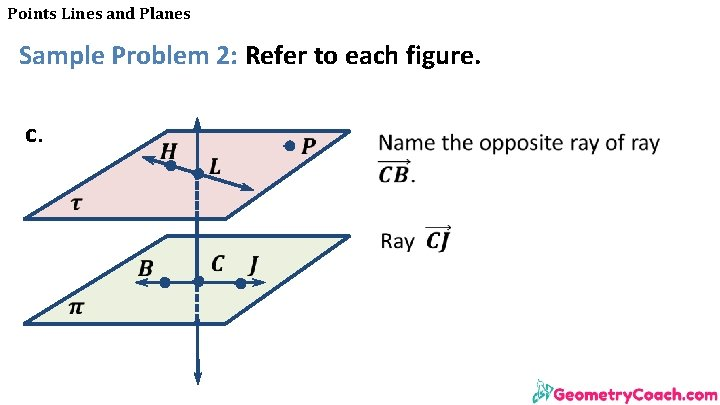Points Lines and Planes Sample Problem 2: Refer to each figure. c.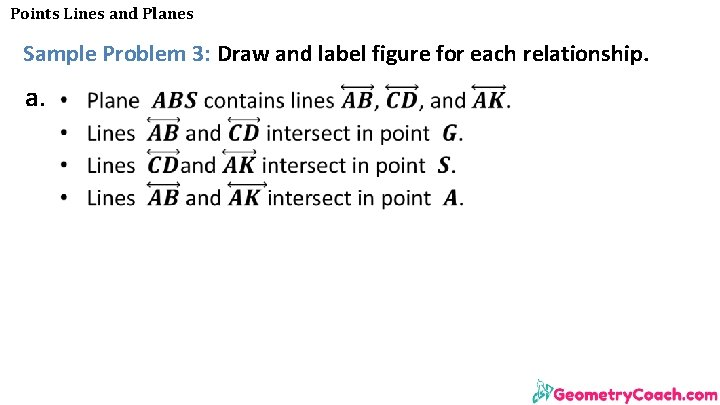Points Lines and Planes Sample Problem 3: Draw and label figure for each relationship. a.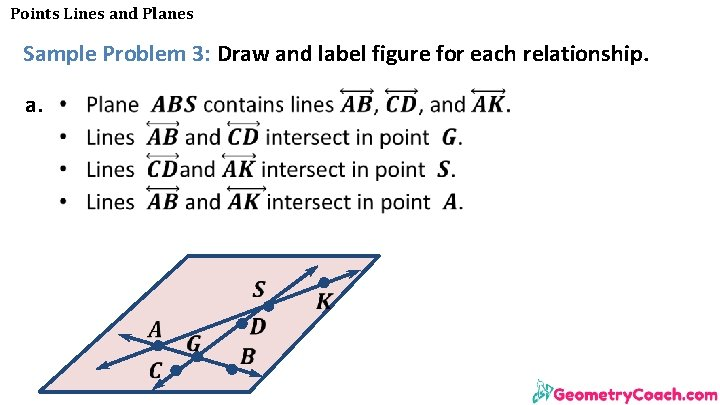Points Lines and Planes Sample Problem 3: Draw and label figure for each relationship. a.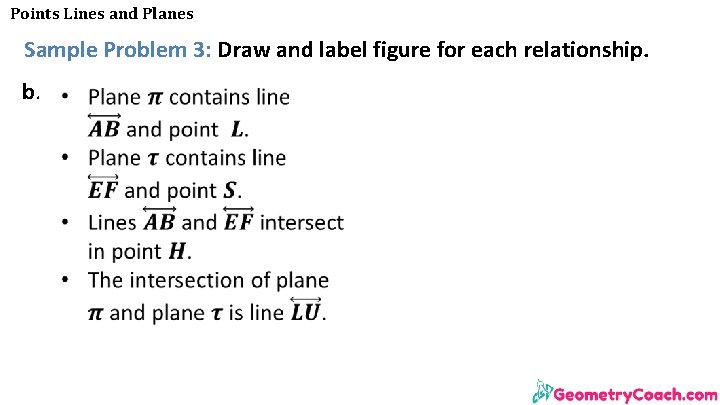Points Lines and Planes Sample Problem 3: Draw and label figure for each relationship. b.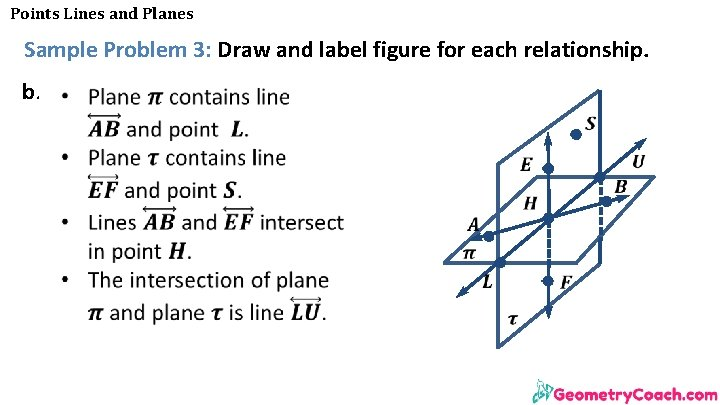Points Lines and Planes Sample Problem 3: Draw and label figure for each relationship. b.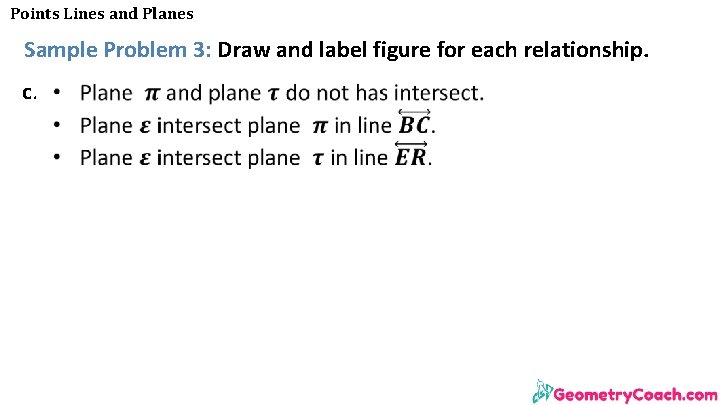Points Lines and Planes Sample Problem 3: Draw and label figure for each relationship. c.Points Lines and Planes Sample Problem 3: Draw and label figure for each relationship. c.